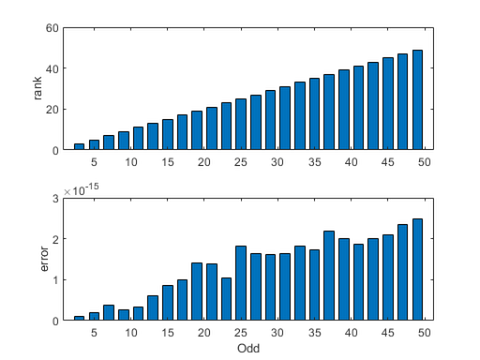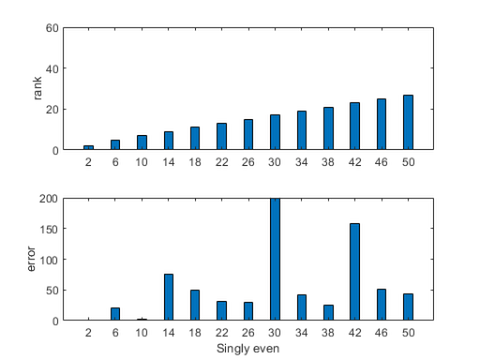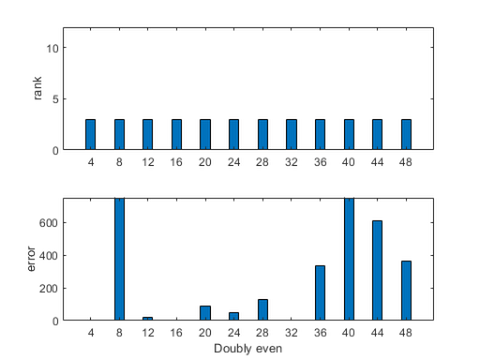# What is A\A?

The answer: A\A is always I, except when it isn't.

### Contents

#### Why A\A ?

I have been explaining our backslash operator for almost 50 years, but I have to admit that the title of today's blog post looks a bit strange. You never see 3\3 for numbers. So, what is A\A ?

A\A solves the equation

   |A*X = A|

If A is square and nonsingular, then X = I. But what if A is rectangular, or singular, or not known exactly? These are all nontrivial questions.

This post is my response to a recent internal discussion at MathWorks about backslash generating NaNs.

#### Mono-elemental matrices

Any general statement about matrices should be applicable to 1-by-1 matrices in particular. For 1-by-1 matrices, there is an easy answer to our question.

• If a is any nonzero number, then a\a = 1.

My colleague Pete Stewart likes to use "mono-elemental" for this important class of matrices.

#### Nonsingular matrices

When the 1-by-1 case is generalized to n-by-n with larger n, it becomes:

• If A is any nonsingular matrix, then A\A = I,

This is not the end of the story, of course. It's our job to investigate approximately nonsingular and approximately equals.

#### Mono-elemental again

When my daughter was in the fifth grade, her math teacher told her that mathematicians hadn't figured out yet how to divide by zero. But the authors of the IEEE 754 standard for floating point arithmetic have figured it out and have assured us that 0\0 is not equal to 1, but rather

• If a = 0, then 0\0 is Not-A-Number.

And, for a diagonal matrix of any order, this scalar case is applicable to each diagonal element.

#### Rank deficient matrices

If A is a rank deficient matrix with rank r < n, then A\A cannot possibly be I. The rank of the product of two matrices cannot be larger than rank of either matrix. So A\A cannot outrank A itself and

• If A is rank deficient, then A\A is definitely not I.

It just so happens that the most recent issue of SIAM Review includes the paper about matrix rank, "LU and CR Elimination", by my colleague Gil Strang and myself. The paper is available from either the SIAM web site or Gil's MIT web site. Another pointer is this Cleve's Corner.

#### Lots of NaNs

Here are three examples where A\A generates NaN .

     A = 0
B = [1 0; 0 0]
C = [1 2; 4 8]

X = A\A
Y = B\B
Z = C\C

A =

0

B =

1     0
0     0

C =

1     2
4     8

X =

NaN

Y =

1     0
NaN   NaN

Z =

NaN   NaN
NaN   NaN



#### Magic squares

I always like to investigate any property of matrices by checking out magic squares.

    small_fig
warning off
nmax = 50;
r = zeros(nmax,1);
e = zeros(nmax,1);

for n  = 1:nmax
A = magic(n);
X = A\A;
I = eye(n);
r(n) = rank(A);
e(n) = norm(X-I);
end


#### Odd

MATLAB uses three different algorithms for computing magic squares, odd, singly even and doubly even. If the order n is odd, then A = magic(n) is nonsingular, the rank of A is n and the elements of the computed A\A are within roundoff error of the elements of I. Notice that the scale factor for the error plot is 3.0e-15.

    n = 3:2:nmax;
plots(n,r,60,e,[],"Odd")#### Singly even

If the order n is divisible by 2, but not by 4, then magic(n) is rank deficient. Its rank is about half its order. The error plot reflects the fact that A\A is not I.

    n = 2:4:nmax;
plots(n,r,60,e,200,"Singly even")#### Doubly even

If the order n is divisible by 4, then magic(n) is very rank deficient. The rank is always 3. The error plots are all over the place. Orders 8 and 40 have errors that are larger than my plot scale. Orders 16 and 32 are missing entirely because computing A\A encounters 0\0 resulting in NaN.

    n = 4:4:nmax;
plots(n,r,12,e,750,"Doubly even")#### Pseudoinverse

Is pinv(A)*b more "robust" than A\b?

You should not use pinv just to create solutions to problems that do not have solutions. The pseudoinverse is intended to characterize the solution to a specific technical question: if a system of linear equations has many solutions, which is the shortest one? If you replace A\b by pinv(A)*b, be sure that is what you want.

Using pinv instead of backslash does not do away with rank deficiency. The difficulties are already present in mono-elemental matrices. The only rank deficient 1-by-1 matrix is 0 and pinv(0) = 0. This is less in-your-face than NaN, but there is no way you can make pinv(0)*0 equal to 1.

When I redo the examples above, I get

     A = 0
B = [1 0; 0 0]
C = [1 2; 4 8]

X = pinv(A)*A
Y = pinv(B)*B
Z = pinv(C)*C

A =

0

B =

1     0
0     0

C =

1     2
4     8

X =

0

Y =

1     0
0     0

Z =

0.2000    0.4000
0.4000    0.8000



The NaNs are gone, but is this really "more robust" than backslash? If you still think so, explain where Z comes from.

#### My Bottom Line

This has been about square, dense, nonsymmetric matrices A. For such matrices:

• A\A may produce NaN for rank-deficient matrices.
• pinv(A)*A avoids NaN by attempting to hide rank-deficient matrices.

Published with MATLAB® R2022a

|